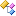﻿ Accord.Statistics.Models.Fields.Functions.Specialized Namespace# Accord.Statistics.Models.Fields.Functions.Specialized NamespaceClasses
ClassDescriptionMarkovDiscreteFactor
Discrete-density Markov Factor Potential (Clique Potential) function.MarkovIndependentFactor
Factor Potential function for a Markov model whose states are independent distributions composed of discrete and Normal distributed components.MarkovMultivariateNormalFactor
Multivariate Normal Markov Model Factor Potential (Clique Potential) function.MarkovNormalFactor
Normal-density Markov Factor Potential (Clique Potential) function.